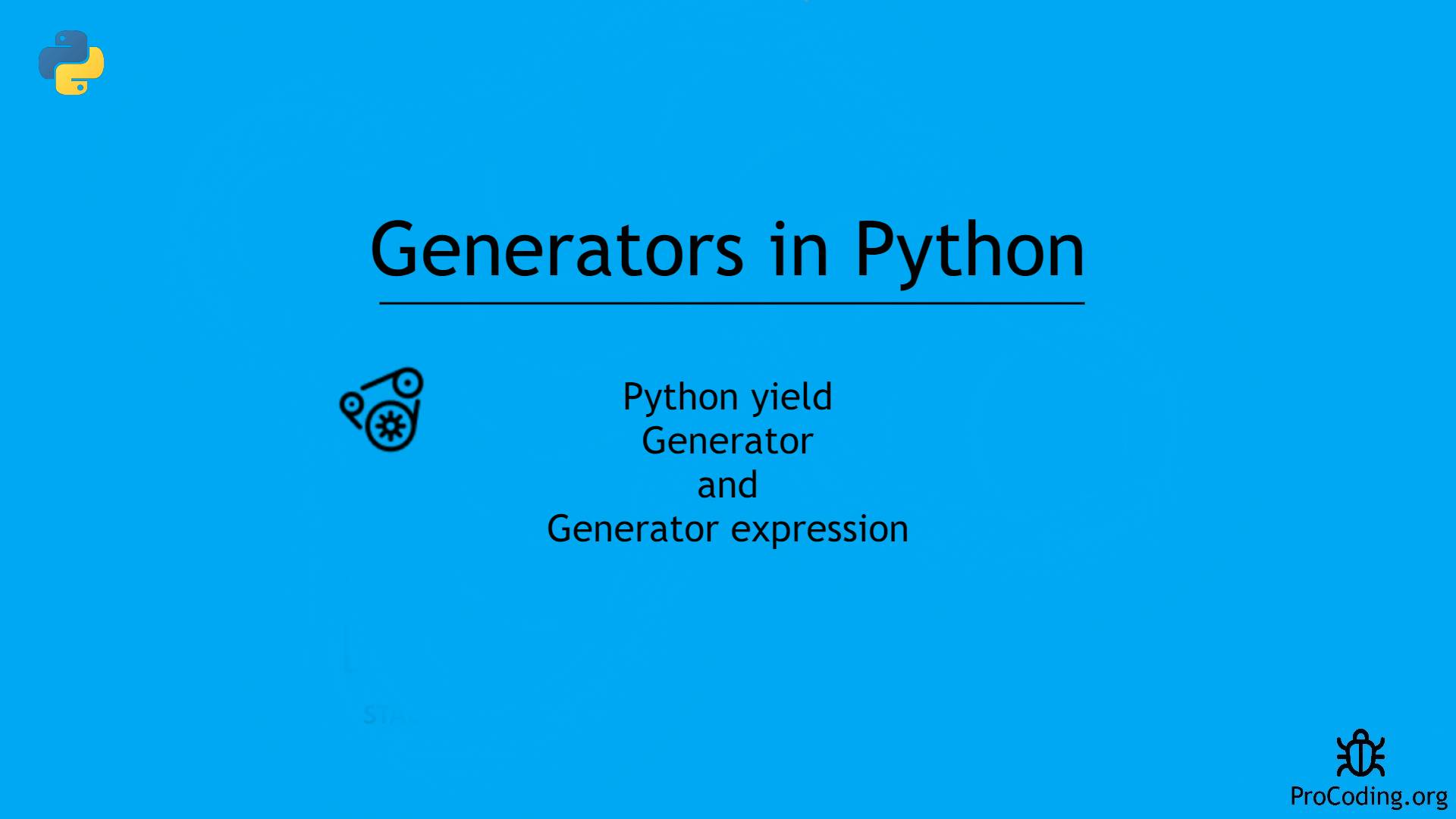# Generators in python: How to use Generators and yield in Python

Category: PythonTags: function python intermediate

A generator is a function which is responsible to generate a sequence of values.
It can be written like a regular function, but it uses the yield keyword.### Generators in Python

Generator is a function that returns an object(iterator) which can be used to get one value at a time.

We can achieve same functionality using iterators also but it is a lengthy process because we have to write `__iter__()` and `__next__()` then raise `StopIteration` exception.

### Writing Generators

Generators are very much similar to function but we use `yield` instead of `return` statement.

We can use any number of `yield` statement in a generator function.

`return` statement terminates the function and controll is passed back to calling function completely. But `yield` statement pauses the function and save its state as well as local variables and continues the execution from that state.
After execution of generator `StopIteration` is raised automatically.

``````def generator():
yield 'a'
yield 'b'
yield 'c'

g = generator()
print(type(g))

print(next(g))
print(next(g))
print(next(g))
print(next(g))``````

Output

```<class 'generator'>
a
b
c
Traceback (most recent call last):
File "demo.py", line 12, in <module>
print(next(g))
StopIteration```

In the example given below you can observe how generator function remembers the state of its local variables.

``````def generator():
num = 1
yield num

num = num + 1
yield num

num = num + 1
yield num

g = generator()

print(next(g))
print(next(g))
print(next(g))
print(next(g))``````

Output

```1
2
3
Traceback (most recent call last):
File "demo.py", line 16, in <module>
print(next(g))
StopIteration```

### Python Generator with loop

Since, generator uses iterator behind the scene we can use `for` loop that takes an iterator and iterate over it using `next()` function and stops the execution when `StopIteration` exception is raised.

``````def generator():
num = 1
yield num

num = num + 1
yield num

num = num + 1
yield num

for num in generator():
print(num)``````

Output

```1
2
3```

Better way to use generator with loop is given below.

Example 1:

``````def firstN(num):
n = 1
while n <= num:
yield n
n = n + 1

for num in firstN(5):
print(num)``````

Output

```1
2
3
4
5```

Example 2:

``````def str_rev(my_str):
length = len(my_str)
for i in range(length - 1, -1, -1):
yield my_str[i]

for char in str_rev("hello"):
print(char)``````

Output

```o
l
l
e
h```

### Creating list using generator function

``````def firstN(num):
n = 1
while n <= num:
yield n
n = n + 1

data = list(firstN(5))
print(data)``````

Output

`[1, 2, 3, 4, 5]`

### Python Generator Expression

In Python, there is a one-liner to create generators which is very much similar to list comprehension.
We have to use round brackets instead of square brackets to create generator evertything else is similar to List comprehension.

Example: Square of numbers using generator Expression

``````nums = [2, 3, 4, 5, 6]
square_num = (x**2 for x in nums)

print(square_num)
for num in square_num:
print(num)``````

Output

```<generator object <genexpr> at 0x000001DF097DCF10>
4
9
16
25
36```

### Performance comparision of Generator with Other Collection

If we compare the performance of generator with other data structure there is a huge difference because data structures performs all tha calculation first and store complete result in the begining but generator performs each operation when it is required and yield the result one by one.

Observe the following example.

``````import random
import time

alpha = ['a', 'b', 'c', 'd', 'e']
nums = [1, 2, 3, 4, 5]

def combination_list(num):
result = []
for i in range(num):
data = {
'id': i,
'alpha': random.choice(alpha),
'num': random.choice(nums)
}
result.append(data)
return result

def combination_generator(num):
for i in range(num):
data = {
'id': i,
'alpha': random.choice(alpha),
'num': random.choice(nums)
}
yield data

t1 = time.clock()
result = combination_list(1000000)
t2 = time.clock()

t3 = time.clock()
result = combination_generator(1000000)
t4 = time.clock()

print('List', t2-t1)
print('Generator', t4-t3)``````

Output

```List 2.7044390000000003
Generator 0.12968809999999964```

### When I should choose Generator?

• While reading large files e.g., csv or excel files which may lead into `MemoryError` if common data structure is used.
• When you have to generate an infinite sequence.

### Advantages of using Generator function

• Generators are easy to use if compared with class level iterators.
• Improves memory utilization and performance.
• Best suitable for reading data from large files.
• Generators works great for web scraping and crawling.